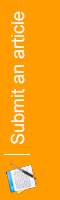Summary: In the work we come across, an information technology course for higher education students, forming the curriculum and the role of information culture in their activity, to choose the curriculum materials, h’s stages and look through them.

Key words: activity, attitude, information, culture, system, teaching, higher education, globalization, university and economics.

Branch of science: Physics and mathematics

Summary: In this article, the application of the MathCAD software package for solving differential equations with partial derivatives is considered.

Key words: mathematics editor, differential equations, partial derivatives.

Branch of science: Physics and mathematics

Abstract: The purpose of this work is to get acquainted with breakthrough technology and equipment for the production of sodium hypochlorite. The pros and cons of other sodium hypochlorite production facilities were considered. As a result, it can be concluded that the proposed technology and equipment can be used.

Branch of science: Physics and mathematics

Summary: There is a numerical and analytical approach to research of stress-strain state of an anisotropic composite finite element field with different boundary conditions on the surface. The problem is solved using a three-dimensional model of the theory of elasticity. The system of differential equations is reduced to one-dimensional problem through the use of spline-collocation in the two coordinate directions. Boundary value problem for a system of ordinary differential equations of higher order is decided with the stable numerical method of discrete orthogonalization.

Key words: stress-strain state, partial differential equations, method of spline collocation, method of discrete orthogonalization, anisotropic finite element region.

Branch of science: Physics and mathematics

Annotation. An infinitely lifting method for making certain types of three-term equations, which is completely refuted by the ABC conjecture.

Keywords: three-term equation, the method of infinite growth, elementary aspect.

Branch of science: Physics and mathematics